Request a Free Counselling Session from our Expert Mentor# NCERT Solution for Class 12 Mathematics Chapter 9

NCERT Solution for Class 12 Mathematics Chapter 9, “Differential Equations,” is an important study material designed to help students understand differential equations’ fundamental concepts and principles. Swastik Classes, a leading coaching institute, has developed comprehensive NCERT solutions that provide step-by-step explanations and solved examples to help students develop a deeper understanding of the subject. The chapter covers the definition of a differential equation, the order and degree of a differential equation, formation of differential equations, and solving differential equations by various methods like separation of variables and homogeneous differential equations. With the help of Swastik Classes’ NCERT solutions, students can improve their problem-solving skills and gain the confidence to tackle complex differential equation problems. These solutions are also useful for students who are preparing for competitive exams like JEE, NEET, and other entrance exams. Overall, Swastik Classes’ NCERT Solution for Class 12 Mathematics Chapter 9 is an essential resource for students who want to excel in mathematics and build a strong foundation in differential equations.

## Answers of Mathematics NCERT solutions for class 12 Chapter 9 Differential Equations

Exercise 9.1

Question 1:

Determine order and degree (if defined) of differential equation

The highest order derivative present in the differential equation is Therefore, its order is four.

The given differential equation is not a polynomial equation in its derivatives. Hence, its degree is not defined.

Question 2:

Determine order and degree (if defined) of differential equation

The given differential equation is:

The highest order derivative present in the differential equation is Therefore, its order is one.

It is a polynomial equation in The highest power raised to is 1. Hence, its degree is one.

Question 3:

Determine order and degree (if defined) of differential equation

The highest order derivative present in the given differential equation is Therefore, its order is two.

It is a polynomial equation in and The power raised to is 1.

Hence, its degree is one.

Question 4:

Determine order and degree (if defined) of differential equation

The highest order derivative present in the given differential equation is Therefore, its order is 2.

The given differential equation is not a polynomial equation in its derivatives. Hence, its degree is not defined.

Question 5:

Determine order and degree (if defined) of differential equation

The highest order derivative present in the differential equation is Therefore, its order is two.

It is a polynomial equation in and the power raised to is 1.

Hence, its degree is one.

Question 6:

Determine order and degree (if defined) of differential equation

The highest order derivative present in the differential equation is Therefore, its order is three.

The given differential equation is a polynomial equation in and

The highest power raised to is 2. Hence, its degree is 2.

Question 7:

Determine order and degree (if defined) of differential equation

The highest order derivative present in the differential equation is Therefore, its order is three.

It is a polynomial equation in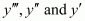. The highest power raised to is 1. Hence, its degree is 1.

Question 8:

Determine order and degree (if defined) of differential equation

The highest order derivative present in the differential equation is Therefore, its order is one.

The given differential equation is a polynomial equation in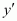and the highest power raised to is one. Hence, its degree is one.

*Question 9:

Determine order and degree (if defined) of differential equation

The highest order derivative present in the differential equation is Therefore, its order is two.

The given differential equation is a polynomial equation in and and the highest power raised to is one.

Hence, its degree is one.

*Question 10:

Determine order and degree (if defined) of differential equation

The highest order derivative present in the differential equation is. Therefore, its order is two.

This is a polynomial equation in and and the highest power raised to is one. Hence, its degree is one.

*Question 11:

The degree of the differential equation

is

(A) 3  (B) 2  (C) 1  (D) not defined

The given differential equation is not a polynomial equation in its derivatives. Therefore, its degree is not defined.

Hence, the correct answer is D.

*Question 12:

The order of the differential equation

is

(A) 2 (B) 1  (C) 0  (D) not defined

The highest order derivative present in the given differential equation is Therefore, its order is two.

Hence, the correct answer is A.

Exercise 9.2

Question 1:

Differentiating both sides of this equation with respect to x, we get:

Now, differentiating equation (1) with respect to x, we get:

Substituting the values of and in the given differential equation, we get the L.H.S. as:

Thus, the given function is the solution of the corresponding differential equation.

Question 2:

Differentiating both sides of this equation with respect to x, we get:

Substituting the value of in the given differential equation, we get: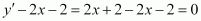L.H.S. =       = R.H.S.

Hence, the given function is the solution of the corresponding differential equation.

Question 3:

Differentiating both sides of this equation with respect to x, we get:

Substituting the value of in the given differential equation, we get:

L.H.S. = R.H.S.

Hence, the given function is the solution of the corresponding differential equation.

Question 4:

Differentiating both sides of the equation with respect to x, we get: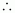L.H.S. = R.H.S.

Hence, the given function is the solution of the corresponding differential equation.

Question 5:

Differentiating both sides with respect to x, we get:

Substituting the value of in the given differential equation, we get:

Hence, the given function is the solution of the corresponding differential equation.

Question 6:

Differentiating both sides of this equation with respect to x, we get:

Substituting the value ofin the given differential equation, we get:

Hence, the given function is the solution of the corresponding differential equation.

Question 7:

Differentiating both sides of this equation with respect to x, we get:L.H.S. = R.H.S.

Hence, the given function is the solution of the corresponding differential equation.

Question 8:

Differentiating both sides of the equation with respect to x, we get:

Substituting the value ofin equation (1), we get:

Hence, the given function is the solution of the corresponding differential equation.

*Question 9:

Differentiating both sides of this equation with respect to x, we get:

Substituting the value of in the given differential equation, we get:

Hence, the given function is the solution of the corresponding differential equation.

*Question 10:

Differentiating both sides of this equation with respect to x, we get:

Substituting the value of in the given differential equation, we get:

Hence, the given function is the solution of the corresponding differential equation.

*Question 11:

The numbers of arbitrary constants in the general solution of a differential equation of fourth order are:

(A) 0  (B) 2  (C) 3  (D) 4

We know that the number of constants in the general solution of a differential equation of order n is equal to its order.

Therefore, the number of constants in the general equation of fourth order differential equation is four.

Hence, the correct answer is D.

*Question 12:

The numbers of arbitrary constants in the particular solution of a differential equation of third order are:

(A) 3 (B) 2 (C) 1 (D) 0

In a particular solution of a differential equation, there are no arbitrary constants.

Hence, the correct answer is D.

Exercise 9.3

Question 1:

Differentiating both sides of the given equation with respect to x, we get:

Again, differentiating both sides with respect to x, we get:

Hence, the required differential equation of the given curve is .

Question 2:

Differentiating both sides with respect to x, we get:

Again, differentiating both sides with respect to x, we get:

Dividing equation (2) by equation (1), we get:

This is the required differential equation of the given curve.

Question 3:

Differentiating both sides with respect to x, we get:

Again, differentiating both sides with respect to x, we get:

Multiplying equation (1) with (2) and then adding it to equation (2), we get:

Now, multiplying equation (1) with 3 and subtracting equation (2) from it, we get:

Substituting the values of  and in equation (3), we get:

This is the required differential equation of the given curve.

Question 4:

Differentiating both sides with respect to x, we get:

Multiplying equation (1) with 2 and then subtracting it from equation (2), we get:

…(3)

Differentiating both sides with respect to x, we get:

y”-2y’ =2be2x                     …(4)

Dividing equation (4) by equation (3), we get:

This is the required differential equation of the given curve.

Question 5:

Differentiating both sides with respect to x, we get:

Again, differentiating with respect to x, we get:

Adding equations (1) and (3), we get:

This is the required differential equation of the given curve.

Question 6:

Form the differential equation of the family of circles touching the y-axis at the origin.

The centre of the circle touching the y-axis at origin lies on the x-axis.

Let (a, 0) be the centre of the circle.

Since it touches the y-axis at origin, its radius is a.

Now, the equation of the circle with centre (a, 0) and radius (a) is

Differentiating equation (1) with respect to x, we get:

Now, on substituting the value of a in equation (1), we get:

This is the required differential equation.

*Question 7:

Form the differential equation of the family of parabolas having vertex at origin and axis along positive y-axis.

The equation of the parabola having the vertex at origin and the axis along the positive y-axis is:

Differentiating equation (1) with respect to x, we get:

Dividing equation (2) by equation (1), we get:

This is the required differential equation.

*Question 8:

Form the differential equation of the family of ellipses having foci on y-axis and centre at origin.

The equation of the family of ellipses having foci on the y-axis and the centre at origin is as follows:

Differentiating equation (1) with respect to x, we get:

Again, differentiating with respect to x, we get:

Substituting this value in equation (2), we get:

This is the required differential equation.

*Question 9:

Form the differential equation of the family of hyperbolas having foci on x-axis and centre at origin.

The equation of the family of hyperbolas with the centre at origin and foci along the x-axis is:

Differentiating both sides of equation (1) with respect to x, we get:

Again, differentiating both sides with respect to x, we get:

Substituting the value of in equation (2), we get:

This is the required differential equation.

*Question 10:

Form the differential equation of the family of circles having centre on y-axis and radius 3 units.

Let the centre of the circle on y-axis be (0, b).

The differential equation of the family of circles with centre at (0, b) and radius 3 is as follows:

Differentiating equation (1) with respect to x, we get:

Substituting the value of (y – b) in equation (1), we get:

This is the required differential equation.

*Question 11:

Which of the following differential equations has as the general solution?

A.  B.  C.  D.

The given equation is:

Differentiating with respect to x, we get:

Again, differentiating with respect to x, we get:

This is the required differential equation of the given equation of curve.

Hence, the correct answer is B.

*Question 12:

Which of the following differential equation has as one of its particular solution?

A.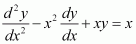B.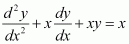C.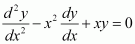D.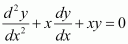The given equation of curve is y = x.

Differentiating with respect to x, we get:

Again, differentiating with respect to x, we get:

Now, on substituting the values of y from equation (1) and (2) in each of the given alternatives, we find that only the differential equation given in alternative C is correct.

Hence, the correct answer is C.

Exercise 9.4

Question 1:

The given differential equation is:

Now, integrating both sides of this equation, we get:

This is the required general solution of the given differential equation.

Question 2:

The given differential equation is:

Now, integrating both sides of this equation, we get:

This is the required general solution of the given differential equation.

Question 3:

The given differential equation is:

Now, integrating both sides, we get:

This is the required general solution of the given differential equation.

Question 4:

The given differential equation is:

Integrating both sides of this equation, we get:

Substituting these values in equation (1), we get:

This is the required general solution of the given differential equation.

Question 5:

The given differential equation is:

Integrating both sides of this equation, we get:

Let (ex + e–x) = t.

Differentiating both sides with respect to x, we get:

Substituting this value in equation (1), we get:

This is the required general solution of the given differential equation.

Question 6:

The given differential equation is:

Integrating both sides of this equation, we get:

This is the required general solution of the given differential equation.

Question 7:

The given differential equation is:

Integrating both sides, we get:

Substituting this value in equation (1), we get:

This is the required general solution of the given differential equation.

Question 8:

The given differential equation is:

Integrating both sides, we get:

This is the required general solution of the given differential equation.

Question 9:

The given differential equation is:

Integrating both sides, we get:

Substituting this value in equation (1), we get:

This is the required general solution of the given differential equation.

Question 10:

The given differential equation is:

Integrating both sides, we get:

Substituting the values of and  in equation (1), we get:

This is the required general solution of the given differential equation.

*Question 11:

The given differential equation is:

Integrating both sides, we get:

Comparing the coefficients of x2 and x, we get:

A + B = 2

B + C = 1

A + = 0

Solving these equations, we get:

Substituting the values of A, B, and C in equation (2), we get:

Therefore, equation (1) becomes:

Substituting C = 1 in equation (3), we get:

*Question 12:

Integrating both sides, we get:

Comparing the coefficients of x2x, and constant, we get:

Solving these equations, we get  and

Substituting the values of AB, and C in equation (2), we get:

Therefore, equation (1) becomes:

Substituting the value of kin equation (3), we get:

*Question 13:

Integrating both sides, we get:

Substituting C = 1 in equation (1), we get:

*Question 14:

Integrating both sides, we get:

Substituting C = 1 in equation (1), we get:

y = sec x

*Question 15:

Find the equation of a curve passing through the point (0, 0) and whose differential equation is

The differential equation of the curve is:

Integrating both sides, we get:

Substituting this value in equation (1), we get:

Now, the curve passes through point (0, 0).

Substituting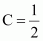in equation (2), we get:

Hence, the required equation of the curve is

*Question 16:

For the differential equation  find the solution curve passing through the point (1, –1).

The differential equation of the given curve is:

Integrating both sides, we get:

Now, the curve passes through point (1, –1).

Substituting C = –2 in equation (1), we get:

This is the required solution of the given curve.

*Question 17:

Find the equation of a curve passing through the point (0, –2) given that at any point  on the curve, the product of the slope of its tangent and y-coordinate of the point is equal to the x-coordinate of the point.

Let and y be the x-coordinate and y-coordinate of the curve respectively.

We know that the slope of a tangent to the curve in the coordinate axis is given by the relation,

According to the given information, we get:

Integrating both sides, we get:

Now, the curve passes through point (0, –2).

∴ (–2)2 – 02 = 2C

⇒ 2C = 4

Substituting 2C = 4 in equation (1), we get:

y2 – x2 = 4

This is the required equation of the curve.

*Question 18:

At any point (xy) of a curve, the slope of the tangent is twice the slope of the line segment joining the point of contact to the point (–4, –3). Find the equation of the curve given that it passes through (–2, 1).

It is given that (xy) is the point of contact of the curve and its tangent.

The slope (m1) of the line segment joining (xy) and (–4, –3) is

We know that the slope of the tangent to the curve is given by the relation,

According to the given information:

Integrating both sides, we get:

This is the general equation of the curve.

It is given that it passes through point (–2, 1).

Substituting C = 1 in equation (1), we get:

y + 3 = (x + 4)2

This is the required equation of the curve.

*Question 19:

The volume of spherical balloon being inflated changes at a constant rate. If initially its radius is 3 units and after 3 seconds it is 6 units. Find the radius of balloon after seconds.

Let the rate of change of the volume of the balloon be k (where k is a constant).

Integrating both sides, we get:

⇒ 4π × 3= 3 (k × 0 + C)

⇒ 108π = 3C

⇒ C = 36π

At = 3, r = 6:

⇒ 4π × 63 = 3 (k × 3 + C)

⇒ 864π = 3 (3k + 36π)

⇒ 3k = –288π – 36π = 252π

⇒ k = 84π

Substituting the values of k and C in equation (1), we get:

Thus, the radius of the balloon after t seconds is .

*Question 20:

In a bank, principal increases continuously at the rate of r% per year. Find the value of r if Rs 100 doubles itself in 10 years (loge 2 = 0.6931).

Let pt, and r represent the principal, time, and rate of interest respectively.

It is given that the principal increases continuously at the rate of r% per year.

Integrating both sides, we get:

It is given that when t = 0, p = 100.

⇒ 100 = ek … (2)

Now, if t = 10, then p = 2 × 100 = 200.

Therefore, equation (1) becomes:

Hence, the value of r is 6.93%.

Question 21:

In a bank, principal increases continuously at the rate of 5% per year. An amount of Rs 1000 is deposited with this bank, how much will it worth after 10 years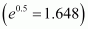.

Let p and t be the principal and time respectively.

It is given that the principal increases continuously at the rate of 5% per year.

Integrating both sides, we get:

Now, when t = 0, p = 1000.

⇒ 1000 = eC … (2)

At t = 10, equation (1) becomes:

Hence, after 10 years the amount will worth Rs 1648.

Question 22:

In a culture, the bacteria count is 1,00,000. The number is increased by 10% in 2 hours. In how many hours will the count reach 2,00,000, if the rate of growth of bacteria is proportional to the number present?

Let y be the number of bacteria at any instant t.

It is given that the rate of growth of the bacteria is proportional to the number present.

Integrating both sides, we get:

Let y0 be the number of bacteria at t = 0.

⇒ log y0 = C

Substituting the value of C in equation (1), we get:

Also, it is given that the number of bacteria increases by 10% in 2 hours.

Substituting this value in equation (2), we get:

Therefore, equation (2) becomes:

Now, let the time when the number of bacteria increases from 100000 to 200000 be t1.

⇒ y = 2y0 at t = t1

From equation (4), we get:

Hence, in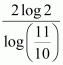hours the number of bacteria increases from 100000 to 200000.

Question 23:

The general solution of the differential equation  is

A.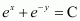B.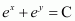C.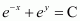D.

Integrating both sides, we get:

Hence, the correct answer is A.

Exercise 9.5

Question 1:

The given differential equation i.e., (x2 + xydy = (x2 + y2dx can be written as:

This shows that equation (1) is a homogeneous equation.

To solve it, we make the substitution as:

vx

Differentiating both sides with respect to x, we get:

Substituting the values of v and  in equation (1), we get:

Integrating both sides, we get:

This is the required solution of the given differential equation.

Question 2:

The given differential equation is:

Thus, the given equation is a homogeneous equation.

To solve it, we make the substitution as:

vx

Differentiating both sides with respect to x, we get:

Substituting the values of y and  in equation (1), we get:

Integrating both sides, we get:

This is the required solution of the given differential equation.

Question 3:

The given differential equation is:

Thus, the given differential equation is a homogeneous equation.

To solve it, we make the substitution as:

vx

Substituting the values of y and  in equation (1), we get:

Integrating both sides, we get:

This is the required solution of the given differential equation.

Question 4:

The given differential equation is:

Therefore, the given differential equation is a homogeneous equation.

To solve it, we make the substitution as:

vx

Substituting the values of y and  in equation (1), we get:

Integrating both sides, we get:

This is the required solution of the given differential equation.

Question 5:

The given differential equation is:

Therefore, the given differential equation is a homogeneous equation.

To solve it, we make the substitution as:

vx

Substituting the values of y and  in equation (1), we get:

Integrating both sides, we get:

This is the required solution for the given differential equation.

Question 6:

Therefore, the given differential equation is a homogeneous equation.

To solve it, we make the substitution as:

vx

Substituting the values of v and  in equation (1), we get:

Integrating both sides, we get:

This is the required solution of the given differential equation.

Question 7:

The given differential equation is:

Therefore, the given differential equation is a homogeneous equation.

To solve it, we make the substitution as:

vx

Substituting the values of y and  in equation (1), we get:

Integrating both sides, we get:

This is the required solution of the given differential equation.

Question 8:

Therefore, the given differential equation is a homogeneous equation.

To solve it, we make the substitution as:

vx

Substituting the values of y and  in equation (1), we get:

Integrating both sides, we get:

This is the required solution of the given differential equation.

Question 9:

Therefore, the given differential equation is a homogeneous equation.

To solve it, we make the substitution as:

vx

Substituting the values of y and  in equation (1), we get:

Integrating both sides, we get:

Therefore, equation (1) becomes:

This is the required solution of the given differential equation.

Question 10:

Therefore, the given differential equation is a homogeneous equation.

To solve it, we make the substitution as:

vy

Substituting the values of x and  in equation (1), we get:

Integrating both sides, we get:

This is the required solution of the given differential equation.

Question 11:

Therefore, the given differential equation is a homogeneous equation.

To solve it, we make the substitution as:

vx

Substituting the values of y and  in equation (1), we get:

Integrating both sides, we get:

Now, y = 1 at x = 1.

Substituting the value of 2k in equation (2), we get:

This is the required solution of the given differential equation.

Question 12:

Therefore, the given differential equation is a homogeneous equation.

To solve it, we make the substitution as:

vx

Substituting the values of y and  in equation (1), we get:

Integrating both sides, we get:

Now, y = 1 at x = 1.

Substituting  in equation (2), we get:

This is the required solution of the given differential equation.

Question 13:

Therefore, the given differential equation is a homogeneous equation.

To solve this differential equation, we make the substitution as:

vx

Substituting the values of y and  in equation (1), we get:

Integrating both sides, we get:

Now,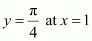.

Substituting C = e in equation (2), we get:

This is the required solution of the given differential equation.

*Question 14:

Therefore, the given differential equation is a homogeneous equation.

To solve it, we make the substitution as:

vx

Substituting the values of y and  in equation (1), we get:

Integrating both sides, we get:

This is the required solution of the given differential equation.

Now, y = 0 at x = 1.

Substituting C = e in equation (2), we get:

This is the required solution of the given differential equation.

*Question 15:

Therefore, the given differential equation is a homogeneous equation.

To solve it, we make the substitution as:

vx

Substituting the value of y and  in equation (1), we get:

Integrating both sides, we get:

Now, y = 2 at x = 1.

Substituting C = –1 in equation (2), we get:

This is the required solution of the given differential equation.

*Question 16:

A homogeneous differential equation of the form  can be solved by making the substitution

A. y = vx

B. v = yx

C. vy

D. x = v

For solving the homogeneous equation of the form , we need to make the substitution as x = vy.

Hence, the correct answer is C.

*Question 17:

Which of the following is a homogeneous differential equation?

A.

B.

C.

D.

Function F(xy) is said to be the homogenous function of degree n, if

F(λx, λy) = λn F(xy) for any non-zero constant (λ).

Consider the equation given in alternative D:

Hence, the differential equation given in alternative D is a homogenous equation.

Exercise 9.6

Question 1:

The given differential equation is

This is in the form of (where p = 2 and Q = sin x)

The solution of the given differential equation is given by the relation,

Therefore, equation (1) becomes:

This is the required general solution of the given differential equation.

Question 2:

The given differential equation is  (where p = 3 and ).

The solution of the given differential equation is given by the relation,

This is the required general solution of the given differential equation.

Question 3:

The given differential equation is:

The solution of the given differential equation is given by the relation,

This is the required general solution of the given differential equation.

Question 4:

The given differential equation is:

The general solution of the given differential equation is given by the relation,

Question 5:

The given differential equation is:

This equation is in the form of:

The general solution of the given differential equation is given by the relation,

Therefore, equation (1) becomes:

Question 6:

The given differential equation is:

This equation is in the form of a linear differential equation as:

The general solution of the given differential equation is given by the relation,

Question 7:

The given differential equation is:

This equation is the form of a linear differential equation as:

The general solution of the given differential equation is given by the relation,

Substituting the value of  in equation (1), we get:

This is the required general solution of the given differential equation.

Question 8:

This equation is a linear differential equation of the form:

The general solution of the given differential equation is given by the relation,

Question 9:

This equation is a linear differential equation of the form:

The general solution of the given differential equation is given by the relation,

Question 10:

This is a linear differential equation of the form:

The general solution of the given differential equation is given by the relation,

Question 11:

This is a linear differential equation of the form:

The general solution of the given differential equation is given by the relation,

Question 12:

This is a linear differential equation of the form:

The general solution of the given differential equation is given by the relation,

Question 13:

The given differential equation is

This is a linear equation of the form:

The general solution of the given differential equation is given by the relation,

Now,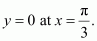Therefore,

Substituting C = –2 in equation (1), we get:

Hence, the required solution of the given differential equation is

*Question 14:

This is a linear differential equation of the form:

The general solution of the given differential equation is given by the relation,

Now, y = 0 at x = 1.

Therefore,

Substituting    in equation (1), we get:

This is the required general solution of the given differential equation.

*Question 15:

The given differential equation is

This is a linear differential equation of the form:

The general solution of the given differential equation is given by the relation,

Now,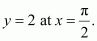Therefore, we get:

Substituting C = 4 in equation (1), we get:

This is the required particular solution of the given differential equation.

*Question 16:

Find the equation of a curve passing through the origin given that the slope of the tangent to the curve at any point (xy) is equal to the sum of the coordinates of the point.

Let F (xy) be the curve passing through the origin.

At point (xy), the slope of the curve will be

According to the given information:

This is a linear differential equation of the form:

The general solution of the given differential equation is given by the relation,

Substituting in equation (1), we get:

The curve passes through the origin.

Therefore, equation (2) becomes:

1 = C

⇒ C = 1

Substituting C = 1 in equation (2), we get:

Hence, the required equation of curve passing through the origin is

*Question 17:

Find the equation of a curve passing through the point (0, 2) given that the sum of the coordinates of any point on the curve exceeds the magnitude of the slope of the tangent to the curve at that point by 5.

Let F (xy) be the curve and let (xy) be a point on the curve. The slope of the tangent to the curve at (xy) is

According to the given information:

This is a linear differential equation of the form:

The general equation of the curve is given by the relation,

Therefore, equation (1) becomes:

The curve passes through point (0, 2).

Therefore, equation (2) becomes:

0 + 2 – 4 = Ce0

⇒ – 2 = C

⇒ C = – 2

Substituting C = –2 in equation (2), we get:

This is the required equation of the curve.

*Question 18:

The integrating factor of the differential equation  is

A. ex

B. ey

C.

D. x

The given differential equation is:

This is a linear differential equation of the form:

The integrating factor (I.F) is given by the relation,

Hence, the correct answer is C.

*Question 19:

The integrating factor of the differential equation.

is

A.

B.

C.

D.

The given differential equation is:

This is a linear differential equation of the form:

The integrating factor (I.F) is given by the relation,

Hence, the correct answer is D.

Miscellaneous Exercise

Question 1:

For each of the differential equations given below, indicate its order and degree (if defined).

(i)

(ii)

(iii)

(i)  The differential equation is given as:

The highest order derivative present in the differential equation is Thus, its order is two. The highest power raised to  is one. Hence, its degree is one.

(ii)  The differential equation is given as:

The highest order derivative present in the differential equation is Thus, its order is one. The highest power raised to  is three. Hence, its degree is three.

(iii)  The differential equation is given as:

The highest order derivative present in the differential equation is Thus, its order is four.

However, the given differential equation is not a polynomial equation. Hence, its degree is not defined.

Question 2:

For each of the exercises given below, verify that the given function (implicit or explicit) is a solution of the corresponding differential equation.

(i)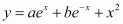Differentiating both sides with respect to x, we get:

Again, differentiating both sides with respect to x, we get:

Now, on substituting the values of  and  in the differential equation, we get:

⇒ L.H.S. ≠ R.H.S.

Hence, the given function is not a solution of the corresponding differential equation.

(ii)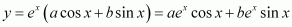Differentiating both sides with respect to x, we get:

Again, differentiating both sides with respect to x, we get:

Now, on substituting the values of  and  in the L.H.S. of the given differential equation, we get:

Hence, the given function is a solution of the corresponding differential equation.

(iii)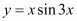Differentiating both sides with respect to x, we get:

Again, differentiating both sides with respect to x, we get:

Substituting the value of  in the L.H.S. of the given differential equation, we get:

Hence, the given function is a solution of the corresponding differential equation.

(iv)

Differentiating both sides with respect to x, we get:

Substituting the value of  in the L.H.S. of the given differential equation, we get:

Hence, the given function is a solution of the corresponding differential equation.

Question 3:

Form the differential equation representing the family of curves given by  where a is an arbitrary constant.

Differentiating with respect to x, we get:

From equation (1), we get:

On substituting this value in equation (3), we get:

Hence, the differential equation of the family of curves is given as

Question 4:

Prove that  is the general solution of differential equation where c is a parameter.

This is a homogeneous equation. To simplify it, we need to make the substitution as:

Substituting the values of y and  in equation (1), we get:

Integrating both sides, we get:

Substituting the values of I1 and I2 in equation (3), we get:

Therefore, equation (2) becomes:

Hence, the given result is proved.

Question 5:

Form the differential equation of the family of circles in the first quadrant which touch the coordinate axes.

The equation of a circle in the first quadrant with centre (aa) and radius (a) which touches the coordinate axes is:

Differentiating equation (1) with respect to x, we get:

Substituting the value of a in equation (1), we get:

Hence, the required differential equation of the family of circles is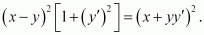Question 6:

Find the general solution of the differential equation

Integrating both sides, we get:

Question 7:

Show that the general solution of the differential equation  is given by (x + + 1) = (1 – – y – 2xy), where is parameter

Integrating both sides, we get:

Hence, the given result is proved.

Question 8:

Find the equation of the curve passing through the point  whose differential equation is,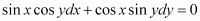The differential equation of the given curve is:

Integrating both sides, we get:

The curve passes through point .

On substituting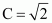in equation (1), we get:

Hence, the required equation of the curve is

Question 9:

Find the particular solution of the differential equation

given that y = 1 when x = 0

Integrating both sides, we get:

Substituting these values in equation (1), we get:

Now, y = 1 at x = 0.

Therefore, equation (2) becomes:

Substituting  in equation (2), we get:

This is the required particular solution of the given differential equation.

Question 10:

Solve the differential equation

Differentiating it with respect to y, we get:

From equation (1) and equation (2), we get:

Integrating both sides, we get:

Question 11:

Find a particular solution of the differential equation given that = – 1, when x = 0 (Hint: put x – y = t)

Substituting the values of x – and  in equation (1), we get:

Integrating both sides, we get:

Now, y = –1 at = 0.

Therefore, equation (3) becomes:

log 1 = 0 – 1 + C

⇒ C = 1

Substituting C = 1 in equation (3) we get:

This is the required particular solution of the given differential equation.

Question 12:

Solve the differential equation

This equation is a linear differential equation of the form

The general solution of the given differential equation is given by,

*Question 13:

Find a particular solution of the differential equation  given that y = 0 when

The given differential equation is:

This equation is a linear differential equation of the form

The general solution of the given differential equation is given by,

Now,

Therefore, equation (1) becomes:

Substituting  in equation (1), we get:

This is the required particular solution of the given differential equation.

*Question 14:

Find a particular solution of the differential equation given that y = 0 when x = 0

Integrating both sides, we get:

Substituting this value in equation (1), we get:

Now, at x = 0 and y = 0, equation (2) becomes:

Substituting C = 1 in equation (2), we get:

This is the required particular solution of the given differential equation.

*Question 15:

The population of a village increases continuously at the rate proportional to the number of its inhabitants present at any time. If the population of the village was 20000 in 1999 and 25000 in the year 2004, what will be the population of the village in 2009?

Let the population at any instant (t) be y.

It is given that the rate of increase of population is proportional to the number of inhabitants at any instant.

Integrating both sides, we get:

log kt + C … (1)

In the year 1999, t = 0 and y = 20000.

Therefore, we get:

log 20000 = C … (2)

In the year 2004, t = 5 and = 25000.

Therefore, we get:

In the year 2009, t = 10 years.

Now, on substituting the values of tk, and C in equation (1), we get:

Hence, the population of the village in 2009 will be 31250.

*Question 16:

The general solution of the differential equation  is

A.  xy = C

B.  = Cy2

C.  = Cx

D.  y = Cx2

The given differential equation is:

Integrating both sides, we get:

Hence, the correct answer is C.

Question 17:

The general solution of a differential equation of the type  is

A.  B.

C.  D.

The integrating factor of the given differential equation is

The general solution of the differential equation is given by,

Hence, the correct answer is C.

*Question 18:

The general solution of the differential equation  is

A.  xey + x2 = C

B.  xey + y2 = C

C.  yex + x2 = C

D.  yey x2 = C

The given differential equation is:

This is a linear differential equation of the form

The general solution of the given differential equation is given by,

Hence, the correct answer is C.

### Key Features of NCERT Solutions for Class 12 Maths Chapter 9- Differential Equations

Learning the chapter Differential Equations using the NCERT Solutions enables the students to understand the following:

Definition, order and degree, general and particular solutions of a differential equation. Formation of differential equation whose general solution is given. Solutions of differential equations by the method of separation of variables, solutions of homogeneous differential equations of first order and first degree. Solutions of linear differential equation of the type:

## Conclusion

Swastik Classes’ NCERT Solution for Class 12 Mathematics Chapter 9, “Differential Equations,” is a comprehensive study material designed to help students understand the fundamental concepts and principles of differential equations. The solutions provide step-by-step explanations and solved examples that help students to develop a deeper understanding of the subject. The chapter covers a range of topics including the definition of a differential equation, order and degree of a differential equation, formation of differential equations, and solving differential equations by various methods like separation of variables and homogeneous differential equations. With the help of these solutions, students can improve their problem-solving skills and gain the confidence to tackle complex differential equation problems. Swastik Classes’ NCERT solutions are designed in accordance with the latest CBSE syllabus, making them useful for students preparing for board exams or competitive exams like JEE and NEET. Overall, Swastik Classes’ NCERT Solution for Class 12 Mathematics Chapter 9 is an excellent resource for students who want to excel in mathematics and build a strong foundation in differential equations.

The principles of Differential Equations are covered in Chapter 9 of NCERT Solutions for Class 12 Maths in an easy-to-understand manner, which aids students in their test preparation.

### Why opt for SWC?

One of the top IIT JEE coaching institutes is Swastik Classes. Shobhit Bhaiya and Alok Bhaiya, pioneering mentors of IIT JEE Coaching Classes, started Swastik Classes in Anand Vihar. Over the last 15 years, they have educated and sent over 2000+ students to IITs and 5000+ students to different famous universities such as BITS, NITs, DTU, and NSIT. When it comes to coaching programmes for IIT JEE, Swastik Classes is the top IIT JEE Coaching in Delhi, favoured by students from all over India.

Swastik Classes’ teachers have a solid academic background, having graduated from IIT with honours, and have extensive expertise in moulding students’ careers.

The study process in Swastik courses is separated between pre-class and post-class work, which is one of the most significant aspects. They are precisely created to improve the student’s mental ability and comprehension.

## FAQs related to NCERT Solutions for Class 12 Math chapter 9 – Differential equation

### What is the formula of differential equations?

dy/dx = f(x)

Partially derivatives and regular derivatives are both included in a differential equation. The differential equation describes a relationship between a variable that is constantly altering with regard to a change in another quantity, and the derivative reflects a rate of change.

### What is a differential equation in simple terms?

A differential equation is a mathematical equation that has one or more derivatives of a function. The function’s derivative is given by dy/dx. To put it another way, it’s an equation that comprises derivatives of one or more dependent variables with regard to one or more independent variables.

### What is a differential equation in calculus?

An equation containing an unknown function y=f(x) and one or more of its derivatives is known as a differential equation. When f and its derivatives are substituted into the equation, a solution to a differential equation is a function y=f(x) that satisfies the differential equation.

### Why is differential equations so hard?

Differential equations is a challenging subject. Differential equations need a solid grasp of preceding concepts like differentiation, integration, and algebraic manipulation. Differential equations are difficult because you must use what you’ve learned in both familiar and novel situations.

### Is differential equations easy?

Don’t be surprised to discover that Differential Equations isn’t quite as tough as many people believe. For 98 percent of ODE (Ordinary Differential Equations), all you need to know is how to integrate.

### What are the applications of differential equations?

In real life, ordinary differential equations are used to compute the movement or flow of electricity, the motion of an item to and fro, such as a pendulum, and to illustrate thermodynamics ideas. In medical terminology, they’re also used to visualise the progression of illnesses in graphical form.

### How do you write a differential equation example?

Consider the differential equation y′=3×2, which has a derivative and is an example of a differential equation. The variables x and y have a relationship: y is an unknown function of x. Furthermore, the derivative of y is on the left-hand side of the equation.